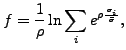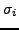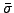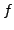Next: *ORIENTATION Up: Input deck format Previous: *NSET   Contents

## *OBJECTIVE

Keyword type: step

With *OBJECTIVE one can define the objective functions in a sensitivity analysis. Right now the following objectives are allowed for TYPE=COORDINATE design variables:

• DISPLACEMENT: the sum of the square of the displacements in all nodes of the structure or of a subset if a node set is defined
• EIGENFREQUENCY: all eigenfrequencies calculated in a previous *FREQUENCY step
• MASS: mass of the total structure or of a subset if an element set is defined
• SHAPEENERGY: internal energy of the total structure or of a subset if an element set is defined
• STRESS: the maximum von Mises stress of the total structure or of a subset if a node set is defined. The maximum is approximated by the Kreisselmeier-Steinhauser function(495)

whereis the von Mises stress in node i,andare user-defined parameters. The higherthe closeris to the actual maximum (a value of 10 is recommended; the higher this value, the sharper the turns in the function).is the target stress, it should not be too far away from the actual maximum.

and for TYPE=ORIENTATION design variables:

• DISPLACEMENT: the displacements in all nodes.
• EIGENFREQUENCY: all eigenfrequencies and eigenmodes calculated in a previous *FREQUENCY step.
• GREEN: the Green functions calculated in a previous *GREEN step.
• MASS: mass of the total structure or of a subset if an element set is defined
• SHAPEENERGY: internal energy of the total structure or of a subset if an element set is defined
• STRESS: the stresses in all nodes.

There are no parameters. Exactly one *OBJECTIVE keyword is required in a *SENSITIVITY step. This keyword has to be followed by at least one objective function. If a *CONSTRAINT card is used within the step exactly one objective function must be defined within the step.

First line:

• *OBJECTIVE.

Second line:

• an objective function
• an element or node set, if appropriate
•for the Kreisselmeier-Steinhauser function (only for the coordinates as design variables and the stress as target)
•for the Kreisselmeier-Steinhauser function (only for the coordinates as design variables and the stress as target)
Repeat this line if needed.

The objective functions SHAPEENERGY, MASS, DISPLACEMENT and STRESS require a *STATIC step before the *SENSITIVITY step, the objective function EIGENFREQUENCY requires a *FREQUENCY step immediately preceding the *SENSITIVITY step and the objective function GREEN requires a *GREEN step before the *SENSITIVITY step. Therefore, the {SHAPEENERGY, MASS, DISPLACEMENT, STRESS} objective functions, the {EIGENFREQUENCY} objective function and the {GREEN} objective function are mutually exclusive within one and the same *SENSITIVITY step.

Example:

*OBJECTIVE
DISPLACEMENT,N1


defines the sum of the square of the displacements in set N1 to be the objective function.

Example files: sensitivity_I.Next: *ORIENTATION Up: Input deck format Previous: *NSET   Contents
guido dhondt 2018-12-15